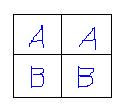## M Theory Lesson 115

As a fellow antipodean (Terence Tao) explains, the decomposition of a function $f: \mathbb{R} \rightarrow \mathbb{R}$ into odd and even parts is the simplest example of a Fourier transform. This is the $2 \times 2$ circulant case, characterised by the identity and the Pauli swap matrix,

01
10

which are both 1-circulants. The swap is associated to reflection about zero on the real line, or rotation by $\pi$ in the complex plane. These small matrices appear too simple to be interesting, but let’s consider a general $2 \times 2$ circulant

A B
B A

Constructing this matrix from row vectors, which in turn are concatenated scalars, may be compared to a construction via a vector of column vectors. We denote these two options by $(A,B)'(B,A)$ and $(A’B),(B’A)$. That these two are equal is an example of the bicategorical interchange law, where the traditional symbolic form of the matrix is literally the 2-arrow diagram! The square of this matrix takes the form

(A.A + B.B) (A.B + B.A)
(B.A + A.B) (B.B + A.A)

which in an interchange diagram subdivides each square into four little squares, introducing two new products (addition and multiplication). Assuming distributivity for the moment, the first entry would satisfy the interchange law only when $A.B + B.A = 0$ and the second entry when $A.A + B.B = 0$. For ordinary numbers this would immediately result in $A = B = 0$, so it might be more interesting to consider this second interchange law to be broken by these terms, the first being the Jordan product.## 2 Responses so far »

1. 1### L. Riofrio said,

Great stuff as always. The simplest example of a Fourier transform (which describes all regular functions) is a Pauli matrix. That in effct quantizes the Fourier transform, reducing wave functions to quantum values.

2. 2### Kea said,

Hi Louise. Yes, wave functions on classical spaces are clumsy affairs. It is much easier to deal with the measurements themselves.